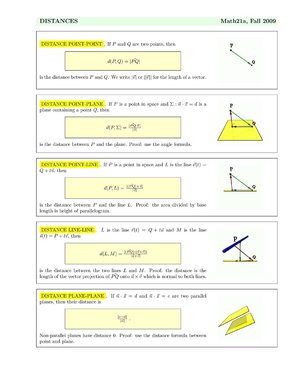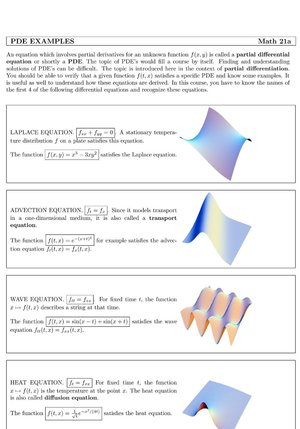6760, Math 21a, Fall 2009
Handouts page of Math 21a, Multivariable Calculus
HandoutsDistance formulas All distance formulas on one page. We expect you to know these formulas or, what is much better, to be able to rederive them.Partial differential equations A short overview over a few partial differential equations. You have to know the Laplace, wave, heat, transport and Burgers equation.